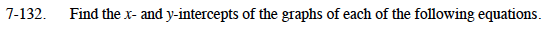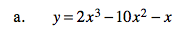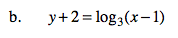### Home > CCA2 > Chapter 7 > Lesson 7.2.2 > Problem7-132

7-132.
1. Find the x- and y-intercepts of the graphs of each of the following equations. Homework Help ✎

1. y = 2x3 − 10x2x

2. y + 2 = log3(x − 1)Set x to 0 and solve for y to find the y-intercept(s).
Set y to 0 and solve for x to find the x-intercept(s).

Let x = 0 to find the y-intercept.

y = 2(0)³ − 10(0)² − (0)

0 = 2x³ − 10x² − x
0 = x(2x² − 10x² − 1)

Let y = 0 to find the x-intercept. Then factor and use the Zero Product Property and the Quadratic Formula.

y-intercept: (0,0)

$x\text{-intercepts: } (0, 0)\text{ and }\left(\frac{5\pm3\sqrt{3}}{2},0\right)$See part (a).

x-intercept: (10, 0)
no y-intercept